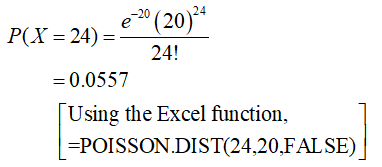The number of different mathematics courses at community colleges has a Poisson Distribution with a mean of 20 courses per college.*show your basic keystrokes and round your answer to 4 decimal places.What is the probability there will be exactly 24 courses at a college?What is the probability there will be fewer than 38 courses at 2 colleges?

Question

The number of different mathematics courses at community colleges has a Poisson Distribution with a mean of 20 courses per college.

*show your basic keystrokes and round your answer to 4 decimal places.

1. What is the probability there will be exactly 24 courses at a college?
2. What is the probability there will be fewer than 38 courses at 2 colleges?
Step 1
1. Probability that there will be exactly 24 courses at a college:

The given information is that the number of different mathematics courses at community colleges has Poisson distribution with mean 20 courses per college.

The probability distribution of a Poisson random variable X with mean λ is given by,

Step 2

Let the random variable X denote the number of different mathematics courses at community colleges. The n, the probability that there will be exactly 24 courses at a college is as obtained as follows:Step 3

Thus, the probability that there will be exactly 24 co...

Want to see the full answer?

See Solution

Want to see this answer and more?

Our solutions are written by experts, many with advanced degrees, and available 24/7

See Solution
Tagged in

Statistics